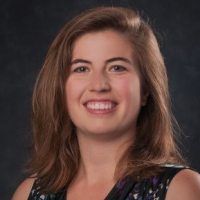# How to mix and match Data

Number

City

1000

A

1100

B

1200

C

1300

D

E

1400

1500

F

1300

Hi Folks,

2 Questions -

1) In the table above, you could see that 1300 is mapped to city 'D' but in last row it is blank.
How do I map it using beast mode or Magic ETL to fill by Lookup in the same column.

Req: Lookup in 'Number' and fill Blanks in 'City'

2) 5th Row contains E in Number and 1400 in City. How do I exchange it to the right position using beast mode or Magic ETL?

Req: Case when 'Number' contains String value then City.
Case when 'City' contains Numeric value then 'Number'

Tagged:

• First, we should solve problem #2. Then, we'll create a canonical lookup table from `Number` to `City` to solve problem #1.

Use an Add Formula tile to make two new columns, `Canonical Number` and `Canonical City` like so:

`Canonical Number` formula: `NULLIF(STR_DIGITS(CASE WHEN City BETWEEN '0' AND '999999' THEN City ELSE Number END), '')`
`Canonical City` formula: `NULLIF(STR_REMOVE_DIGITS(CASE WHEN Number BETWEEN 'A' AND 'zzzzzz' THEN Number ELSE City END), '')`

Now use a Group By tile to make the lookup table. Our one grouping column will be `Canonical Number`, and our one aggregate will be the First non-null value of `Canonical City`; we'll call this the `Lookup City`.

Now use the Join Data tile to Inner Join the original table (the output of the Add Formula) to the lookup table (the output of the Group By) on the `Canonical Number` column.

The `Canonical Number` column and the `Lookup City` column in the result of the Join Data tile have the final values you want.

The whole transform has this shape:

• First, we should solve problem #2. Then, we'll create a canonical lookup table from `Number` to `City` to solve problem #1.

Use an Add Formula tile to make two new columns, `Canonical Number` and `Canonical City` like so:

`Canonical Number` formula: `NULLIF(STR_DIGITS(CASE WHEN City BETWEEN '0' AND '999999' THEN City ELSE Number END), '')`
`Canonical City` formula: `NULLIF(STR_REMOVE_DIGITS(CASE WHEN Number BETWEEN 'A' AND 'zzzzzz' THEN Number ELSE City END), '')`

Now use a Group By tile to make the lookup table. Our one grouping column will be `Canonical Number`, and our one aggregate will be the First non-null value of `Canonical City`; we'll call this the `Lookup City`.

Now use the Join Data tile to Inner Join the original table (the output of the Add Formula) to the lookup table (the output of the Group By) on the `Canonical Number` column.

The `Canonical Number` column and the `Lookup City` column in the result of the Join Data tile have the final values you want.

The whole transform has this shape:

•CoachYou could recreate those two fields as follows and then drag them into your card in place of the current 2 fields:

• City_2: CASE WHEN `Number` = 1300 THEN 'D' ELSE `City` END
• Number_2: CASE WHEN `Number` = 'E' THEN 1400 ELSE `Number` END

•Coach@Rupak I second @colemenwilson's recommendation of recreating the fields. I would do this in MagicETL using the Formula Tile. If you don't want to specify every value to swap, you could use a regular expression to match patterns based on whether` `Number`` contains alpha characters and ``City` `contains numeric characters.
City_2: `CASE WHEN REGEXP_LIKE(`Number`,'[a-zA-Z].*]') then `Number` else `City` END`
Number_2: `CASE WHEN REGEXP_LIKE(`City`,'[0-9].*') then `City` else `Number` END`